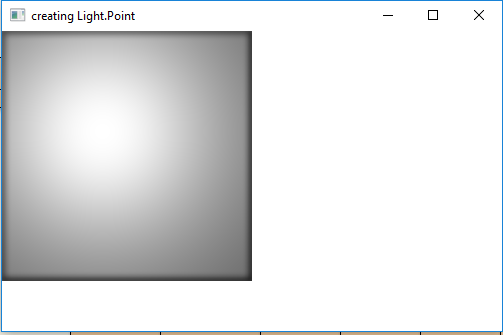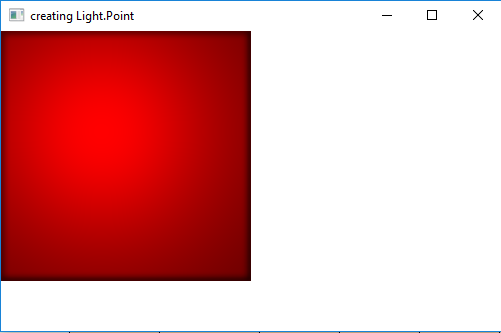GeeksforGeeks App
Open AppBrowser
Continue

Light.Point class is a part of JavaFX. Light.Point class represents a point light source in 3D space. Light.Point class extends the Light class.

Constructors of the class:

1. Point(): Creates a new Point Light object with default values.
2. Point(double x, double y, double z, Color color): Creates a new Point Light object with x, y, z and color values.

Commonly Used Methods:

MethodExplanation
getX()Returns the value of x.
getY()Returns the value of y.
getZ()Returns the value of z.
setX(double v)Sets the value of x.
setY(double v)Sets the value of y.
setZ(double v)Sets the value of z.
getColor()Returns the color of light.
setColor(Color v)Sets the color of light.

Below programs illustrate the use of Light.point class:

1. Java Program to create a Point light and add it to a rectangle: In this program we will create a Rectangle named rectangle with specified height and width. We will also create a Light.Point object named light. We will set the x, y, z values using setX(), setY() and setZ() function. Now create a lighting object and add the light object to lighting using setLight() function. We will set the Lighting effect to the Rectangle and add it to the scene and add the scene to the stage and call the show function to display the results.

 `// Java Program to create a Point light ``// and add it to a rectangle``import` `javafx.application.Application;``import` `javafx.scene.Scene;``import` `javafx.scene.shape.Rectangle;``import` `javafx.scene.control.*;``import` `javafx.stage.Stage;``import` `javafx.scene.Group;``import` `javafx.scene.effect.Light.*;``import` `javafx.scene.effect.*;``import` `javafx.scene.paint.Color;`` ` `public` `class` `Point_1 ``extends` `Application {`` ` `    ``// launch the application``    ``public` `void` `start(Stage stage)``    ``{`` ` `        ``// set title for the stage``        ``stage.setTitle(``"creating Light.Point"``);`` ` `        ``// create point Light object``        ``Light.Point light = ``new` `Light.Point();`` ` `        ``// set coordinates``        ``light.setX(``100``);``        ``light.setY(``100``);``        ``light.setZ(``100``);`` ` `        ``// create a lighting``        ``Lighting lighting = ``new` `Lighting();`` ` `        ``// set Light of lighting``        ``lighting.setLight(light);`` ` `        ``// create a rectangle``        ``Rectangle rect = ``new` `Rectangle(``250``, ``250``);`` ` `        ``// set fill``        ``rect.setFill(Color.WHITE);`` ` `        ``// set effect``        ``rect.setEffect(lighting);`` ` `        ``// create a Group``        ``Group group = ``new` `Group(rect);`` ` `        ``// create a scene``        ``Scene scene = ``new` `Scene(group, ``500``, ``300``);`` ` `        ``// set the scene``        ``stage.setScene(scene);`` ` `        ``stage.show();``    ``}`` ` `    ``// Main Method``    ``public` `static` `void` `main(String args[])``    ``{`` ` `        ``// launch the application``        ``launch(args);``    ``}``}`

Output:2. Java Program to create a Point light and add it to a rectangle and set the color of the light to red: In this program we will create a Rectangle named rectangle with specified height and width. We will also create a Light.Point object named light. Now pass the x, y, z and the color values as parameters of the constructor. We will create a lighting object and add the light object to lighting using setLight() function. We will set the Lighting effect to the Rectangle and add it to the scene and add the scene to the stage and call the show function to display the results.

 `// Java Program to create a Point light and add it to``// a rectangle and set the color of the light to red``import` `javafx.application.Application;``import` `javafx.scene.Scene;``import` `javafx.scene.shape.Rectangle;``import` `javafx.scene.control.*;``import` `javafx.stage.Stage;``import` `javafx.scene.Group;``import` `javafx.scene.effect.Light.*;``import` `javafx.scene.effect.*;``import` `javafx.scene.paint.Color;`` ` `public` `class` `Point_2 ``extends` `Application {`` ` `    ``// launch the application``    ``public` `void` `start(Stage stage)``    ``{`` ` `        ``// set title for the stage``        ``stage.setTitle(``"creating Light.Point"``);`` ` `        ``// create point Light object``        ``Light.Point light = ``new` `Light.Point(``100``, ``100``, ``                                     ``100``, Color.RED);`` ` `        ``// create a lighting``        ``Lighting lighting = ``new` `Lighting();`` ` `        ``// set Light of lighting``        ``lighting.setLight(light);`` ` `        ``// create a rectangle``        ``Rectangle rect = ``new` `Rectangle(``250``, ``250``);`` ` `        ``// set fill``        ``rect.setFill(Color.WHITE);`` ` `        ``// set effect``        ``rect.setEffect(lighting);`` ` `        ``// create a Group``        ``Group group = ``new` `Group(rect);`` ` `        ``// create a scene``        ``Scene scene = ``new` `Scene(group, ``500``, ``300``);`` ` `        ``// set the scene``        ``stage.setScene(scene);`` ` `        ``stage.show();``    ``}`` ` `    ``// Main Method``    ``public` `static` `void` `main(String args[])``    ``{`` ` `        ``// launch the application``        ``launch(args);``    ``}``}`

Output:Note: The above programs might not run in an online IDE. Please use an offline compiler.

My Personal Notes arrow_drop_up
Related Tutorials